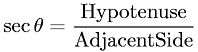Equations > Trigonometry > Trigonometric Definitions > Secant Definition for a Right Triangle

### Secant Definition for a Right TriangleLatex Code:

MathML Code:

 $\mathrm{sec}\theta =\frac\mathrm{Hypotenuse}\mathrm{Adjacent}\mathrm{Side}$

MathType 5.0: